# EMF Equation of DC Generator

The derivation of EMF equation for DC generator has two parts:

1. Induced EMF of one conductor
2. Induced EMF of the generator

## Derivation for Induced EMF of One Armature Conductor

For one revolution of the conductor,
Let,
Φ = Flux produced by each pole in weber (Wb)
and
P = number of poles in the DC generator.

therefore,
Total flux produced by all the poles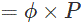And,
Time taken to complete one revolution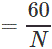Where,
N = speed of the armature conductor in rpm.
Now, according to Faraday’s law of induction, the induced emf of the armature conductor is denoted by “e” which is equal to rate of cutting the flux.

Therefore,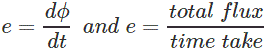Induced emf of one conductor is
Induced emf of one conductor is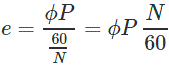## Derivation for Induced EMF for DC Generator

Let us suppose there are Z total numbers of conductor in a generator, and arranged in such a manner that all parallel paths are always in series.
Here,
Z = total numbers of conductor
A = number of parallel paths
Then,
Z/A = number of conductors connected in series
We know that induced emf in each path is same across the line
Therefore,
Induced emf of DC generator
E = emf of one conductor × number of conductor connected in series.

Induced emf of DC generator is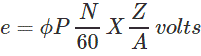Simple wave wound generator
Numbers of parallel paths are only 2 = A
Therefore,
Induced emf for wave type of winding generator is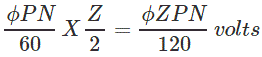Simple lap-wound generator
Here, number of parallel paths is equal to number of conductors in one path
i.e. P = A
Therefore,
Induced emf for lap-wound generator is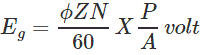Want To Learn Faster? 🎓
Get electrical articles delivered to your inbox every week.
No credit card required—it’s 100% free.